# Introducing Automation: Learn to Automate Data Preparation with Python LibrariesIn this blog we are discussing automation, a function for automating data preparation using a mix of Python libraries. So let’s start.

#### Problem statement

A data containing the following observation is given to you in which the first row contains column headers and all the other rows contains the data. Some of the rows are faulty, a row is faulty if it contains at least one cell with a NULL value. You are supposed to delete all the faulty rows containing NULL value written in it.

In the table given below, the second row is faulty, it contains a NULL value in salary column. The first row is never faulty as it contains the column headers. In the data provided to you every cell in a column may contain a single word and each word may contain digits between 0 & 9 or lowercase and upper case English letters. For example: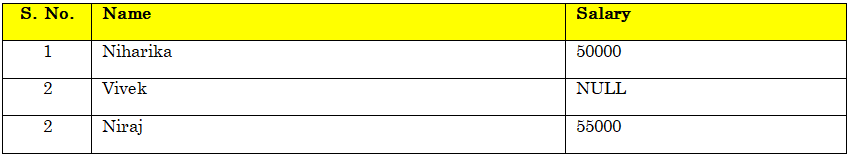In the above example after removing the faulty row the table looks like this: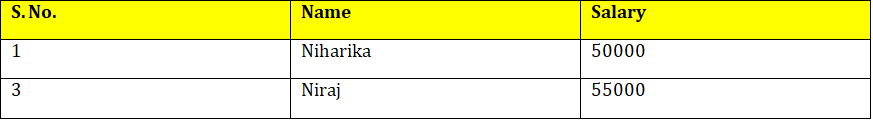The order of rows cannot be changed but the number of rows and columns may differ in different test case.

The data after preparation must be saved in a CSV format. Every two successive cells in each row are separated by a single comma ‘,’symbol and every two successive rows are separated by a new-line ‘\n’ symbol. For example, the first table from the task statement to be saved in a CSV format is a single string ‘S. No., Name, Salary\n1,Niharika,50000\n2,Vivek,NULL\n3,Niraj,55000’ . The only assumption in this task is that each row may contain same number of cells.

Write a python function that converts the above string into the given format.

#### Write a function:

def Solution(s)

Given a string S of length N, returns the table without the Faulty rows in a CSV format.

Given S=‘S. No., Name, Salary\n1,Niharika,50000\n2,Vivek,NULL\n3,Niraj,55000’

The table with data from string S looks as follows: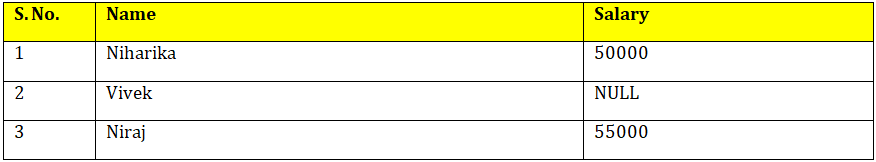After removing the rows containing the NULL values the table should look like this: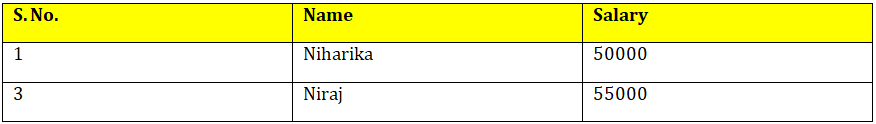You can try a number of strings to cross-validate the function you have created.

Let’s begin.

In the above code the list is converted to an array and then used to create a dataframe and stored as csv file in the default working directory.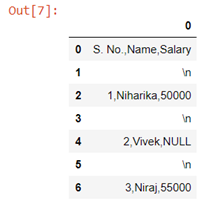The above code creates a dataframe with multiple columns.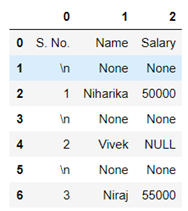Now after dropping the rows with NaN values data looks like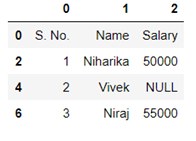To reset the index we can now use .reset_index() method.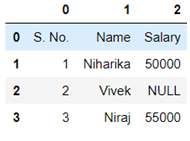Now we will be able to drop the NaN values easily by using .dropna() method.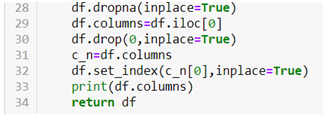In the above code we first dropped the NaN values  then we used the first row of the data set to create column names and then dropped the original row. We also made the first column as index.

Hence we have managed to create a function that can give us the above data. Once created this function can be used to convert a string into dataframe with similar pattern.

Hopefully, you found the discussion informative enough. For further clarification watch the video attached below the blog. To access more informative blogs on Data science using python training related topics, keep on following the Dexlab Analytics blog.

Here’s a video introduction to Automation. You can check it down below to develop a considerable understanding of the same:

.

Comments are closed here.### Call us to know more### Gurgaon+91 931 572 5902